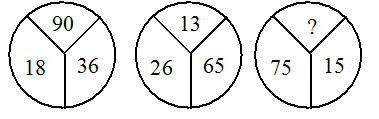A figure is given which is divided into certain parts. Each part bears a number, alphabet or both and one part is blank. Numbers/alphabets follow a certain pattern or rule. So you are required to analyse the given figures, decipher the rule / pattern and then fill the blank space with the appropriate answer

# A figure is given which is divided into certain parts. Each part bears a number, alphabet or both and one part is blank. Numbers/alphabets follow a certain pattern or rule. So you are required to analyse the given figures, decipher the rule / pattern and then fill the blank space with the appropriate answer1. A

30

2. B

75

3. C

45

4. D

60

Fill Out the Form for Expert Academic Guidance!l

+91

Live ClassesBooksTest SeriesSelf Learning

Verify OTP Code (required)

### Solution:+91

Live ClassesBooksTest SeriesSelf Learning

Verify OTP Code (required)